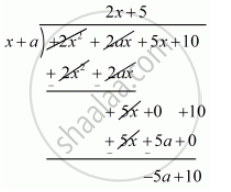Advertisement Remove all ads

# If (X + A) is a Factor of 2x2 + 2ax + 5x + 10, Find A. - Mathematics

Short Note
Sum

If (x + a) is a factor of 2x2 + 2ax + 5x + 10, find a.

Advertisement Remove all ads

#### Solution

Given  (x + a) is a factor of f(x) = 2x^2 + 2ax + 5x + 10.

Let us now divide  f(x) by x + a .

We have,Now, remainder = 0

 10 - 5a = 0

 10 = 5a

10/5=a

2 =a

Hence, the value of a is  2 .

Is there an error in this question or solution?
Advertisement Remove all ads

#### APPEARS IN

RD Sharma Class 10 Maths
Chapter 2 Polynomials
Q 28 | Page 60
Advertisement Remove all ads

#### Video TutorialsVIEW ALL 

Advertisement Remove all ads
Share
Notifications

View all notifications

Forgot password?